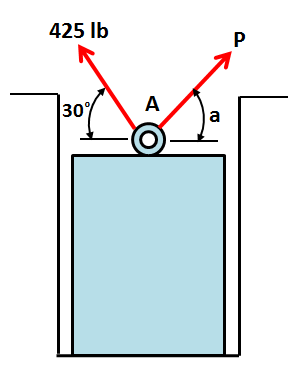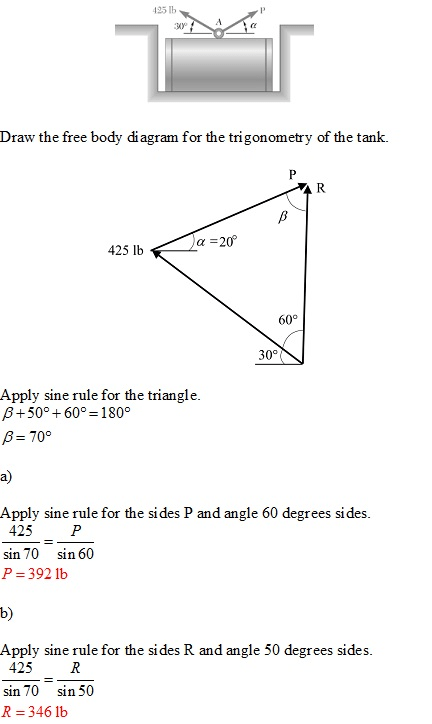# A steel tank is to be positioned in an excavation. Knowing that α=20∘, determine by trigonometry (a) the required magnitude of the force P if the resultant R of the two forces applied at A is to be vertical, (b) the corresponding magnitude of R.

Question-AnswerCategory: Engineering MechanicsA steel tank is to be positioned in an excavation. Knowing that α=20∘, determine by trigonometry (a) the required magnitude of the force P if the resultant R of the two forces applied at A is to be vertical, (b) the corresponding magnitude of R.

### A steel tank is to be positioned in an excavation. Knowing that α=20∘, determine by trigonometry (a) the required magnitude of the force P if the resultant R of the two forces applied at A is to be vertical, (b) the corresponding magnitude of R.